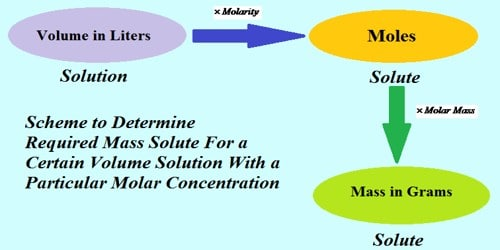Chemistry

# Decimolar Solution is a Standard SolutionDecimolar solution is a standard solution

The solution whose strength is known accurately is called standard solution. Again decimolar solution is that solution when a one-tenth mole of a solute remains in a one-litre solution that means the strength of decimolar solution is known. So decimolar solution is called a standard solution. Decimolar solution means that the molarity of the solution is 1/10.

e.g: 0.1 M NaOH solution means that the strength of NaOH is 0.1M which is known. So, 0.1 M NaOH solutions are a standard solution. So, Decimolar solution is that solution when a one-tenth mole of a solute remains in a one-litre solution that means the strength of decimolar solution is known.